• 原文地址：Matlab图像处理函数汇总 作者：mimi 图像的变换  ① fft2：fft2函数用于数字图像的二维傅立叶变换，如：i=imread('104_8.tif'); j=fft2(i); ②ifft2:：ifft2函数用于数字图像的二维傅立叶反变换，如...

原文地址：Matlab图像处理函数汇总

作者：mimi

图像的变换
j=fft2(i);
②ifft2:：ifft2函数用于数字图像的二维傅立叶反变换，如：
j=fft2(i);
k=ifft2(j);

噪声生成函数
① imnoise：用于对图像生成模拟噪声，如：
j=imnoise(i,'gaussian',0,0.02);%模拟高斯噪声

定义滤波器
② fspecial：用于产生预定义滤波器，如：
h=fspecial('sobel');%sobel水平边缘增强滤波器
h=fspecial('gaussian');%高斯低通滤波器
h=fspecial('laplacian');%拉普拉斯滤波器
h=fspecial('log');%高斯拉普拉斯（LoG）滤波器
h=fspecial('average');%均值滤波器

图像的增强
①直方图：imhist函数用于数字图像的直方图显示，如：
imhist(i);
②直方图均化：histeq函数用于数字图像的直方图均化，如：
j=histeq(i);
④对数变换：log函数用于数字图像的对数变换，如：
j=double(i);
k=log(j);
h=[1,2,1;0,0,0;-1,-2,-1];
j=filter2(h,i);
⑥线性滤波：利用二维卷积conv2滤波, 如:
h=[1,1,1;1,1,1;1,1,1];
h=h/9;
j=conv2(i,h);
⑦中值滤波：medfilt2函数用于图像的中值滤波，如：
j=medfilt2(i);
⑧锐化
（1）利用Sobel算子锐化图像, 如:
h=[1,2,1;0,0,0;-1,-2,-1];%Sobel算子
j=filter2(h,i);
（2）利用拉氏算子锐化图像, 如:
j=double(i);
h=[0,1,0;1,-4,0;0,1,0];%拉氏算子
k=conv2(j,h,'same');
m=j-k;

图像边缘检测
①sobel算子 如：
j = edge(i,'sobel',thresh)
②prewitt算子 如：
j = edge(i,'prewitt',thresh)
③roberts算子  如：
j = edge(i,'roberts',thresh)
④log算子  如：
j = edge(i,'log',thresh)
⑤canny算子 如：
j = edge(i,'canny',thresh)
⑥Zero-Cross算子 如：
j = edge(i,'zerocross',thresh)

形态学图像处理
①膨胀：是在二值化图像中“加长”或“变粗”的操作，函数imdilate执行膨胀运算，如：
b=[0 1 0;1 1 1;0 1 0];
c=imdilate(a,b);
②腐蚀：函数imerode执行腐蚀，如：
b=strel('disk',1);
c=imerode(a,b);
③开运算：先腐蚀后膨胀称为开运算，用imopen来实现，如：
b=strel('square',2);
c=imopen(a,b);
④闭运算：先膨胀后腐蚀称为闭运算，用imclose来实现，如：
b=strel('square',2);
c=imclose(a,b);

===========================================================
Matlab 图像处理相关函数命令大全
一、通用函数：
colorbar  显示彩色条
语法：colorbar colorbar('vert') colorbar('horiz') colorbar(h) h=colorbar(...) colorbar(...,'peer',axes_handle)
getimage 从坐标轴取得图像数据
语法：A=getimage(h) [x,y,A]=getimage(h) [...,A,flag]=getimage(h) [...]=getimage

imshow 显示图像
语法：imshow(I,n) imshow(I,[low high]) imshow(BW) imshow(X,map) imshow(RGB) imshow(...,display_option) imshow(x,y,A,...) imshow filename h=imshow(...)

montage 在矩形框中同时显示多幅图像
语法：montage(I) montage(BW) montage(X,map) montage(RGB) h=montage(...)

immovie 创建多帧索引图的电影动画
语法：mov=immovie(X,map) mov=immovie(RGB)

subimage 在一副图中显示多个图像
语法：subimage(X,map) subimage(I) subimage(BW)   subimage(RGB) subimage(x,y,...) subimage(...)

truesize 调整图像显示尺寸
语法：truesize(fig,[mrows mcols]) truesize(fig)

warp 将图像显示到纹理映射表面
语法：warp(X,map) warp(I ,n) warp(z,...) warp(x,y,z,...)   h=warp(...)

zoom 缩放图像
语法：zoom on zoom off zoom out zoom reset zoom zoom xon zoom yon zoom(factor) zoom(fig,option)

二、图像文件I/O函数命令
imfinfo  返回图形图像文件信息
语法：info=imfinfo(filename,fmt) info=imfinfo(filename)

imwrite  把图像写入（保存）图像文件中
语法：imwrite(A,filename,fmt) imwrite(X,map,filename,fmt) imwrite(...,filename) imwite(...,Param1,Val1,Param2,Val2...)

imcrop  剪切图像
语法：I2=imcrop(I) X2=imcrop(X,map) RGB2=imcrop(RGB) I2=imcrop(I,rect) X2=imcrop(RGB,rect) [...]=imcrop(x,y,...) [A,rect]=imcrop(...) [x,y,A,rect]=imcrop(...)

imresize  改变图像大小
语法：B=imresize(A,m,method)

imrotate  旋转图像
语法：B=imrotate(A,angle,method) B=imrotate(A,angle,method,'crop')

三、像素和统计处理函数
corr2  计算两个矩形的二维相关系数
语法：r=corr2(A,B)

imcontour 创建图像数据的轮廓图
语法：imcontour(I,n) imcontour(I,v) imcontour(x,y,...) imcontour(...,LineSpec) [C,h] =imcontour(...)

imfeature  计算图像区域的特征尺寸
语法：stats=imfeature(L,measurements) stats=imfeature(L,measurements,n)

imbist  显示图像数据的柱状图

impixel 确定像素颜色值
语法：P=impixel(I) P=impixel(X,map) P=impixel(RGB) P=impixel(I,c,r) P=impixel(X,map,c,r) P=impixel(RGB,c,r) [c,r,P]=impixel(...) P=impixel(x,y,I,xi,yi) P=impixel(x,y,RGB,xi,yi) P=impixel(x,y,X,map,xi,yi)
[xi,yi,P]=impixel(x,y,...)

improfile 沿线段计算剖面图的像素值
语法：c=improfile c=improfile(n) c=improfile(I,xi,yi) c=improfile(I,xi,yi,n)   [cx,cy,c]=improfile(...) [cx,cy,c,xi,yi]=improfile(...) [...]=improfile(x,y,I,xi,yi)   [...]=improfile(x,y,I,xi,yi,n)
[...]=improfile(...,method)

mean2 计算矩阵元素的平均值
语法：B=mean2(A)

pixval  显示图像像素信息
语法：pixval on

std2 计算矩阵元素的标准偏移
语法：b=std2(A)

四、图像分析函数：
edge 图像边缘检测
语法：BW=edge(I,'sobel') BW=edge(I,'sobel',thresh) BW=edge(I,'sobel',thresh,direction) [BW,thresh]=edge(I,'sobel',...) BW=edge(I,'prewitt') BW=edge(I,'prewitt',thresh) BW=edge(I,'prewitt',thresh,direction)
[BW,thresh]=edge(I,'prewitt',...)   BW=edge(I,'roberts') BW=edge(I,'roberts',thresh) [BW,thresh]=edge(I,'roberts',...) BW=edge(I,'log') BW=edge(I,'log',thresh) BW=edge(I,'log',thresh,sigma) [BW,threshold]=edge(I,'log',...)
BW=edge(I,'zerocross',thresh,h) [BW,thresh]=edge(I,'zerocross',...)
BW=edge(I,'canny') BW=edge(I,'canny',thresh) BW=edge(I,'canny',thresh,sigma) [BW,threshold]=edge(I,'canny',...)
qtgetblk  获取四叉树分解的块值
语法：[vals,r,c]=qtgetblk(I,S,dim) [vals,idx]=qtgetblk(I,S,dim)
qtsetblk 设置四叉树分解中的块值
语法：J=qtsetblk(I,S,dim,vals)

五、图像增强函数
histeq 用柱状图均等化增强对比
语法：J=histeq(I,hgram) J=histeq(I,n) [J,T]=histeq(I,...) newmap=histeq(X,map,hgram) newmap=histeq(X,map)
imnoise 增强图像的渲染效果
语法：J=imnoise(I,type) J=imnoise(I,type,parameters)
medfilt2 进行二维中值过滤
语法：B=medfilt2(A,[m n]) B=medfilt2(A) B=medfilt2(A,'indexed',...)
ordfilt2 进行二维统计顺序过滤
wiener2 进行二维适应性去噪过滤处理
语法：J=wiener2(I,[m  n],noise) [J,noise]=wiener2(I,[m n])

六、线性滤波函数
conv2 进行二维卷积操作
语法：C=conv2(A,B) C=conv2(hcol,hrow,A) C=conv2(...,'shape')
convmtx2 计算二维卷积矩阵
语法：T=convmtx2(H,m,n) T=convmtx2(H,[m n])
convn 计算n维卷积
语法：C=convn(A,B) C=convn(A,B,'shape')
filter2 进行二维线性过滤操作
语法：Y=filter2(h,X) Y=filter2(h,X,shape)
fspecial 创建预定义过滤器
语法：h=fspecial(type) h=fspecial(type,parameters)

七、线性二维滤波设计函数
freqspace 确定二维频率响应的频率空间
语法：[f1,f2]=freqspace(n) [f1,f2]=freqspace([m n]) [x1 ,y1]=freqspace(...,'meshgrid') f=freqspace(N) f=freqspace(N,'whole')
freqz2 计算二维频率响应
语法：[H,f1,f2]=freqz2(h,n1,n2) [H,fi,f2]]=freqz2(h,[n2,n1]) [H,fi,f2]]=freqz2(h,f1,f2]) [H,fi,f2]]=freqz2(h) [...]=freqz2(h,...,[dx dy]) [...]=freqz2(h,...,dx) freqz2(...)
fsamp2 用频率采样法设计二维FIR过滤器
语法：h=fsamp2(Hd) h=fsamp2(f1,f2,Hd,[m n])
ftrans2 通过频率转换设计二维FIR过滤器
语法：h=ftrans2(b,t) h=ftrans2(b)
fwind1 用一维窗口方法设计二维FIR过滤器
语法：h=fwind1(Hd,win) h=fwind1(Hd,win1,win2) h=fwind1(f1,f2,Hd,...)
fwind2 用二维窗口方法设计二维FIR过滤器
语法：h=fwind2(Hd,win) h=fwind2(f1,f2,Hd,win)

八、图像变换函数
dct2 进行二维离散余弦变换(反余弦变换用idct2)
语法：B=dct2(A) B=dct2(A,m.n) B=dct2(A,[m n])
dctmtx 计算离散余弦傅立叶变换
语法：D=dctmtx(n)
fft2 进行二维快速傅立叶变换(反变换用ifft2)
语法：Y=fft2(X) Y=fft2(X,m,n)
fftn 进行n维快速傅立叶变换(反变换用ifftn)
语法：Y=ffn(X) Y=fftn(X,siz)
fftshift 快速傅立叶变换的DC组件移到光谱中心
语法：Y=fftshift(X) Y=fftshift(X,dim)
phantom 产生一个头部幻影图像
语法：P=phantom(def,n) P=phantom(E,n) [P,E]=phantom(...)

九、边沿和块处理函数
bestblk 确定进行块操作的块大小
语法：siz=bestblk([m n],k) [mb,nb]=bestblk([m n],k)
blkproc 实现图像的显示块操作
语法：B=blkproc(A,[m n]),fun) B=blkproc(A,[m n],fun,P1,P2,...) B=blkproc(A,[m n],[mborder nborder],fun,...)
col2im 将矩阵的列重新组织到块中
语法：A=col2im(B,[m n],[mm nn],block_type) A=col2im(B,[m n],[mm nn])
colfilt 利用列相关函数进行边沿操作
语法：B=colfilt(A,[m n],block_type,fun) B=colfilt(A,[m n],block_type,fun,P1,P2,...) B=colfilt(A,[m n],[mblock nblock],...) B=colfilt(A,'indexed',...)
im2col 重调图像块为列
语法：B=im2col(A,[m n],block_type) B=im2col(A,[m n]) B=im2col(A,'indexed',...)
nlfilter 进行边沿操作
语法：B=nlfilter(A,[m n],fun) B=nlfilter(A,[m n],fun,P1,P2,...) B=nlfilter(A,'indexed',...)

十、二进制图像操作函数
applylut 在二进制图像中利用lookup表进行行边沿操作
语法：A=applylut(BW,LUT)
bwarea 计算二进制图像对象的面积
语法：total=bwarea(BW)
bweuler 计算二进制图像的欧拉数
语法：eul=bweuler(BW)
bwfill 填充二进制图像的背景色
语法：BW2=bwfill(BW1,c,r,n) BW2=bwfill(BW1,n) [BW2,idx]=bwfill(...) BW2=bwfill(x,y,BW1,xi,yi,n) [x,y,BW2,idx,xi,yi]=bwfill(...)   [BW2,idx]=bwfill(BW1,'holes',n)
bwlabel 标注二进制图像中已连接的部分
语法：L=bwlabel(BW,n) [L,num]=bwlabel(BW,n)
bwmorph 提取二进制图像的轮廓
语法：BW2=bwmorph(BW1,operation) BW2=bwmorph(BW1,operation,n)
bwperim 计算二进制图像中对象的周长
语法：BW2=bwperim(BW1) BW2=bwperim(BW1,CONN)
bwselect 在二进制图像中选择对象
语法：BW2=bwselect(BW1,c,r,n) BW2=bwselect(BW1,n) [BW2,idx]=bwselect(...) BW2=bwselect(x,y,BW1,xi,yi,n) [x,y,BW2,idx,xi,yi]=bwselect(...)
dilate 放大二进制图像
语法：BW2=dilate(BW1,SE) BW2=dilate(BW1,SE,alg) BW2=dilate(BW1,SE,...,n)
erode 弱化二进制图像的边界
语法：BW2=erode(BW1,SE) BW2=erode(BW1,SE,alg) BW2=erode(BW1,SE,...,n)
makelut 创建一个用于applylut函数的lookup表
语法：lut=makelut(fun,n) lut=makelut(fun,n,P1,P2,...)

十一、区域处理函数
roicolor 选择感兴趣的颜色区
语法:BW=roicolor(A,low,high) BW=rocicolor(A,v)
roifill 在图像的任意区域中进行平滑插补
语法:J=roifill(I,c,r) J=roifill(I) J=roifill(I,BW) [J,BW]=roifill(...) J=roifill(x,y,I,xi,yi) [x,y,J,BW,xi,yi]=roifill(...)
roifilt2 过滤敏感区域
语法:J=roifilt2(h,I,BW) J=roifilt2(I,BW,fun) J=roifilt2(I,BW,fun,P1,P2,...)
roipoly 选择一个敏感的多边形区域
语法:BW=roipoly(I,c,r) BW=roipoly(I) BW=roipoly(x,y,I,xi,yi) [BW,xi,yi]=roipoly(...) [x,y,BW,xi,yi]=roipoly(...)

十二、颜色映像处理函数
brighten 增加或降低颜色映像表的亮度
语法：brighten(beta) brighten(h,beta) newmap=brighten(beta) newmap=brighten(cmap,beta)
cmpermute 调整颜色映像表中的颜色
语法：[Y,newmap]=cmpermute(X,map) [Y,newmap]=cmpermute(X,map,index)
cmunigue 查找颜色映像表中特定的颜色及相应的图像
语法：[Y,newmap]=cmunigue(X,map) [Y,newmap]=cmunigue(RGB) [Y,newmap]=cmunique(I)
imapprox 对索引图像进行近似处理
语法：[Y,newmap]=imapprox(X,map,n)   [Y,newmap]=imapprox(X,map,tol) Y=imapprox(X,map,newmap) [...]=imapprox(...,dither_option)
rgbplot 划分颜色映像表
语法：rgbplot(cmap)

十三、颜色空间转换函数
hsv2rgb 转换HSV值为RGB颜色空间：M=hsv2rgb(H)
ntsc2rgb 转换NTSC值为RGB颜色空间：rgbmap=ntsc2rgb(yiqmap) RGB=ntsc2rgb(YIQ)
rgb2hsv 转换RGB值为HSV颜色空间：cmap=rgb2hsv(M)
rgb2ntsc 转换RGB值为NTSC颜色空间：yiqmap=rgb2ntsc(rgbmap) YIQ=rgb2ntsc(RGB)
rgb2ycbcr 转换RGB值为YCbCr颜色空间：ycbcrmap=rgb2ycbcr(rgbmap) YCBCR=rgb2ycbcr(RGB)
ycbcr2rgb 转化YCbCr值为RGB颜色空间：rgbmap=ycbcr2rgb(ycbcrmap) RGB=ycbcr2rgb(YCBCR)

十四、图像类型和类型转换函数
dither 通过抖动增加外观颜色分辨率转换图像
语法：X=dither(RGB,map) BW=dither(I)
gray2ind 转换灰度图像为索引图像
语法：[X,map]=gray2ind(I,n) [X,map]=gray2ind(BW,n)
grayslice 从灰度图像为索引图像
语法：X=grayslice(I,n) X=grayslice(I,v)
im2bw 转换图像为二进制图像
语法：BW=im2bw(I,level) BW=im2bw(X,map,level) BW=im2bw(RGB,level)
im2double 转换图像矩阵为双精度型
语法：I2=im2double(I1) RGB2=im2double(RGB1) I=im2double(BW) X2=im2double(X1,'indexed')
double 转换数据为双精度型
语法：double(X)
unit8 、unit16转换数据为8位、16位无符号整型： i=unit8(x) i=unit16(x)
im2unit8 转换图像阵列为8位无符号整型
语法：I2=im2unit8(I1) RGB2=im2unit8(RGB1) I=im2unit8(BW) X2=im2unit8(X1,'indexed')
im2unit16 转换图像阵列为16位无符号整型
语法：I2=im2unit16(I1) RGB2=im2unit16(RGB1) I=im2unit16(BW) X2=im2unit16(X1,'indexed')
ind2gray 把检索图像转化为灰度图像
语法：I=ind2gray(X,map)
ind2rgb  转化索引图像为RGB真彩图像
语法：RGB=ind2rgb(X,map)
isbw 判断是否为二进制图像
语法：flag=isbw(A)
isgray 判断是否为灰度图像
语法：flag=isgray(A)
isind 判断是否为索引图像
语法：flag=isind(A)
isrgb 判断是否为RGB真彩色图像
语法：flag=isrgb(A)
mat2gray 转换矩阵为灰度图像
语法：I=mat2gray(A,[amin amax]) I=mat2gray(A)
rgb2gray 转换RGB图像或颜色映像表为灰度图像
语法：I=rgb2gray(RGB) newmap=rgb2gray(map)
rgb2ind 转换RGB图像为索引图像
语法：[X,map]=rgb2ind(RGB,tol) [X,map]=rgb2ind(RGB,n) X=rgb2ind(RGB,map) [...]=rgb2ind(...,dither_option)

十五、新增图像处理工具箱函数
applycform 用于颜色空间变换 out=applyform(I,C)
bwboundaries 描绘二进制图像边界
语法： B=bwboundaries(BW) B=bwboundaries(BW,CONN) B=bwboundaries(BW,CONN,options) [BW,CONN,options] [BL]=bwboundaries(...) [BLNA]=bwboundaries()
bwtraceboundary 描述二进制图像中的物体
B=bwtraceboundary(BW,P,fstep) B=bwtraceboundary(BW,P,fstep,CONN) B=bwtraceboundary(...N,dir)
decorrstrech 对多通道图像进行去相关处理
语法：S=decorrstretch(I) S=decorrstretch(I,TOL)
dicomdict 获取或读取DICOM文件
语法：dicomdict('set',dictionary) dictionary=dicomdict('get')
getline 用鼠标选择ployline
语法：[x,y]=getline(fig) [x,y]=getline(ax) [x,y]=getline [x,y]=getline(...,'closed')
getpts 用鼠标选择像素点
语法：[x,y]=getpts(fig) [x,y]=getpts(ax) [x,y]=getpts
getrect 用鼠标选择矩阵
语法：rect=getrect(fig) rect=getrect(ax) rect=getrect(fig)
im2java2d 将图像转换为Java缓冲图像
语法：jimage=im2java2d(I) jimage=im2java2d(X,MAP)
imview 在图像与蓝旗中显示图像
语法：imview(I)   imview(RGB) imview(X,map) imview(I,range) imview(filename) imview(....'InitialMagnification',initial_mag) h=imview(...)    imview close all
ippl 检查IPPL的存在
语法：TF=ippl [TF B]=ippl
iptdemos 显示图像处理工具箱中的索引图像
lab2double、lab2unit16、lab2unit8 将L*a*b数据分别转换为双精度、16位数据、8位数据
makecform 创造一个色彩转换结构
unitlut 查找表中A像素值
语法：B=unitlut(A,LUT)
xyz2double、xyz2unit16 将颜色数据从XYZ转换到双精度、16进制。
语法：xyzd=xyz2double(XYZ) xyz16=xyz2unit16(xyz)


展开全文图像处理 matlab 编程 数据 函数
• 原文地址：基于MATLAB图像处理程序（全文转载）作者：星夜图像变换(傅立叶变换), 图像增强, 边缘检测, 滤波, 图像压缩等. 实验工具:MATLAB软件 课程设计时间:2008年12月 实　验　部　分 1.   图像变换 程序代码...

原文地址：基于MATLAB的图像处理程序（全文转载）作者：星夜图像变换(傅立叶变换), 图像增强, 边缘检测, 滤波, 图像压缩等.
实验工具:MATLAB软件
课程设计时间:2008年12月
实　验　部　分

1.
图像变换
程序代码及说明
clear all
N=100;

f=zeros(50,50);
%产生一个50*50的全0数组

f(15:35,23:28)=1;

%定义图像数组，从15行到35行，23列到28列附值为1，为白色，其他区域为黑色

figure(1)
%创建窗口的图形对象，句柄为1

imshow(f,'notruesize')
%显示图像f
F=fft2(f,N,N);
%在二维傅立叶变换前把f截断或者添加0，使其成为N*N的数组
F2=fftshift(abs(F));
%把傅立叶变换的零频率部分移到频谱的中间
figure(2)

x=1:N;y=1:N;
%定义x和y的范围

mesh(x,y,F2(x,y));colormap(gray);colorbar

%绘制立体网状图，将图形对象的色度改为灰度图像，colorbar给坐标轴添加色彩条

%构建一个类似于figure(1)的矩形函数
N=200;
f=zeros(100,100);

f(30:70,45:55)=1;

%定义图像数组，从30行到70行，45列到55列附值为1，为白色，其他区域为黑色
imshow(f,'notruesize');

%然后对f进行二维快速傅立叶变换：以下列出你自己编写的代码…
N=200;
f=zeros(100,100);
f(30:70,45:55)=1;
imshow(f,'notruesize');
title('原始图像');

F=fft2(f,N,N);
%对图像f进行二维快速傅立叶变换
grid
on
%打开网格线
axis
on
%打开坐标轴

imshow(F,[-1,5],'notruesize');
%显示傅立叶变换后的图像，图像数据的值域为[-1,5]
x=1:N;y=1:N;
title('二维快速傅立叶变换后的图像');

mesh(abs(F));
%绘制F的频谱图
title('傅立叶变换后的频谱图');
%然后对上述二维快速傅立叶变换提高分辨率：

要提高二维快速傅立叶变换的分辨率，在采样率一定的情况下，增大采
样点数N即可。对应的频谱图见测试结果。
N=300;
f=zeros(100,100);
f(30:70,45:55)=1;
figure(1)
imshow(f,'notruesize');
title('原始图像');
F=fft2(f,N,N);
axis on
figure(2)
imshow(F,[-1,5],'notruesize');
title('二维快速傅立叶变换后的图像');
x=1:N;y=1:N;
figure(3)
mesh(abs(F));
title('傅立叶变换后的频谱图');

N=400;
f=zeros(100,100);
f(30:70,45:55)=1;
figure(1)
imshow(f,'notruesize');
title('原始图像');
F=fft2(f,N,N);
axis on
figure(2)
imshow(F,[-1,5],'notruesize');
title('二维快速傅立叶变换后的图像');
x=1:N;y=1:N;
figure(3)
mesh(abs(F));
title('傅立叶变换后的频谱图');
�系数移动（使用函数fftshift）

N=200;
f=zeros(100,100);
f(30:70,45:55)=1;
figure(1)
imshow(f,'notruesize');
title('原始图像');
F=fft2(f,N,N);
axis on
figure(2)
imshow(F,[-1,5],'notruesize');
title('二维快速傅立叶变换后的图像');
x=1:N;y=1:N;
figure(3)

mesh(fftshift(abs(F)));
%把零频率部分移到频谱的中间
title('傅立叶变换后的频谱图');
%滤波器频率响应

x=1:N;y=1:N;

mesh(x,y,F2(x,y));colormap(gray);colorbar

%绘制立体网状图，将图形对象的色度改为灰度图像，colorbar给坐标轴添加色彩条

测试结果图：

1》对f进行二维快速傅立叶变换
（请自己运行查看）

2》对上述二维快速傅立叶变换提高分辨率

N=300时：
N=400时：

N=800时：
N=1000时：
（请自己运行查看）

从傅立叶变换的频谱图中可以看出，提高分辨率以后，其边缘更加平滑，锯齿状明显减弱。但其傅立叶变换后的图像没有明显改变。
3》DC系数移动
其系数移动以后，频谱分量都集中到了频谱的中间。
4》滤波器频率响应
（请自己运行查看）

2. 图像增强

图像增强是指按特定的需要突出一幅图像中的某些信息，同时，消弱或去除某些不需要的信息的处理方法。其主要目的是使处理后的图像对某些特定的应用比原来的图像更加有效。图像增强技术主要包含直方图修改处理、图像平滑化处理、图像尖锐化处理、和彩色处理技术等。图像增强有图像对比度增强、亮度增强，轮廓增强等等。
下面利用直方图统计算法对灰度图像进行增强：
程序代码：
subplot(121)
imshow(I);
title('原始图像');
subplot(122)

imhist(I,64)

%绘制图像的直方图，n=64为灰度图像灰度级，若I为灰度图像，默认n=256；
若I为二值图像，默认n=2。
title('图像的直方图');
（请自己运行查看）
n=256时：
（请自己运行查看）
下面利用直方图均衡化增强图像的对比度：

J=histeq(I);

%将灰度图像转换成具有64（默认）个离散灰度级的灰度图像
imshow(I)
title('原始图像')
figure,imshow(J)
title('直方图均衡化后的图像')
figure(1)
subplot(121);imhist(I,64)
title('原始图像的直方图')
subplot(122);imhist(J,64)
title('均衡化的直方图')

（请自己运行查看）

分析：从上图中可以看出，用直方图均衡化后，图像的直方图的灰度间隔被拉大了，均衡化的图像的一些细节显示了出来，这有利于图像的分析和识别。直方图均衡化就是通过变换函数histeq将原图的直方图调整为具有“平坦”倾向的直方图，然后用均衡直方图校正图像。

下面利用直方图规定化对图像进行增强：
figure,imshow(I);
title('原始图像');

hgram=50:2:250;
%规定化函数
J=histeq(I,hgram);
figure,imshow(J);
title('直方图规定化后的图像');
figure,imhist(I,64);
title('原始图像的直方图');
figure,imhist(J,64);
title('直方图规定化后的直方图');
运行结果：
（请自己运行查看）

变换灰度间隔后的图像和直方图：

hgram=50:1:250;
hgram=50:5:250;

3. 图像重建

图像重建的最典型的应用是医学上的计算机断层摄影技术（CT技术）。它用于人体头部、腹部等内部器官的无损伤诊断，其基本方法就是根据人体截面投影，经过计算机处理来重建截面图像。在人体中把需要扫描的部分取出一定厚度的断层面，再把断层面分成许多小的方块。当一束较窄的射线通过每个方块后强度就有一定程度的衰减，衰减的量由此方块的分子构成和组织密度决定。如果通过各种角度重复上述过程以获得一系列强度分布曲线，就有可能从这些数据中计算每一方块的衰减量。这样就能够重建断层或三维图像。
目前提出的图像重建方法有以下五种：
（1）联立方程法（也称矩阵法）；
（2）逆投影法；
（3）付立叶变换法；
（4）滤波──逆投影法（也称卷积法）；
（5）逐次逼近法。

滤波──逆投影法是当前用得较多的一种图像重建方法，在当代射线CT系统中几乎都用这种方法构成系统。它的特点是精度高，能快速实现。

利用R各列中投影值来构造图像I的近似值。投影数越多，获得的图像越接近原始图像，角度theta必须是固定增量的均匀向量。

程序代码：

P=phantom(256);
%用phantom函数产生Sheep-Logan的大脑图，n为图像p中的行列数，默认为256
imshow(P)
title('原始图像')
%以下为三种不同角度的投影模式

%存在18个角度投影

%存在36个角度投影

%存在90个角度投影

figure,imagesc(theta3,xp,R3);colormap(hot);colorbar;

xlabel('theta');ylabel('xprime');
%定义坐标轴
figure,imshow(I1)
figure,imshow(I2)
figure,imshow(I3)

运行结果图：

（请自己运行查看）

由上面重建的图像中可以看出，只用18个投影来重建图像效果很差，而36个投影来重建的图像要好的多，90个投影来重建的图像质量更好，失真也很小，由于R1重建图像的投影太少，所以存在许多虚假点，重建的效果与投影数目相关，投影数目越多图像重建的效果越好，所以要提高重建图像的质量，就需要增加投影角度的数目。

除此之外，还可以在Fan-Beam变换数据中用ifaanbeam函数重建图像。

4. 滤波
4.1 目的
运用中值滤波克服线性滤波器所带来的图像细节模糊。
4.2 使用设备

PC兼容机一台，操作系统为Windows2000（或Windows98，WindowsXP，以下默认为Windows2000）
4.3 使用滤波对图像进行增强

4.3.1
线性滤波（邻域平均）

线性低通滤波器最常用的是线性平滑滤波器，这种滤波器的所有系数都是正的，也称邻域平均。邻域平均减弱或消除了傅立叶变换的高频分量，对噪声的消除有所增强，但是由于平均而使图像变得更为模糊，细节的锐化程度逐渐减弱。
下面使用不同的平滑模板对图像进行滤波：（二维线性滤波fliter2）
程序代码：
imshow(I)
title('原始图像')
J=imnoise(I,'salt &
pepper');
%添加盐椒噪声，噪声密度为默认值0.05
figure,imshow(J)
title('添加盐椒噪声后的图像')

K1=filter2(fspecial('average',3),J)/255;
%应用3×3邻域窗口法
figure,imshow(K1)
title('3×3窗的邻域平均滤波图像')

K2=filter2(fspecial('average',7),J)/255;
%应用7×7邻域窗口法
figure,imshow(K2)
title('7×7窗的邻域平均滤波图像')

K3=filter2(fspecial('average',9),J)/255;
%应用9×9邻域窗口法
figure,imshow(K3)
title('9×9窗的邻域平均滤波图像')

K4=filter2(fspecial('average',11),J)/255;
%应用11×11邻域窗口法
figure,imshow(K4)
title('11×11窗的邻域平均滤波图像')
运行结果图：

（请自己运行查看）

4.3.2 中值滤波

中值滤波可以保留目标边缘，这是中值滤波器相对于均值滤波器的最大优势。中值滤波具有去噪的性能，可以消除孤立的噪声点，可以用来减弱随机干扰和脉冲干扰，但是边缘不模糊。
程序代码：
imshow(I)
title('原始图像')
J=imnoise(I,'salt &
pepper',0.02);  %添加盐椒噪声，噪声密度为0.02
figure,imshow(J)
title('添加盐椒噪声后的图像')

K1=medfilt2(J);
%在默认的3×3的邻域窗中进行中值滤波
figure,imshow(K1)
title('默认的3×3的邻域窗的中值滤波图像')
K2=medfilt2(J,[5
5]);
%在5×5的邻域窗中进行中值滤波
figure,imshow(K2)
title('5×5的邻域窗的中值滤波图像')
运行结果图：
（请自己运行查看）

从上可见，中值滤波的效果要比邻域平均的低通滤波效果好，中值滤波以后的图像的轮廓比较清晰，而且使用较小的模板得到的视觉效果反而好一些。

4.3.3 锐化滤波

图像锐化处理的目的是使模糊图像变得清晰，锐化滤波器减弱或消除了傅立叶空间的低频分量，保留高频分量，从而加强了图像的轮廓，使图像看起来比较清晰。
下面应用Laplacian算子对图像进行锐化处理：
Laplacian算子是线性二次微分算子，其格式为：h =
fspecial('laplacian',
alpha)，返回一个3×3的滤波器来近似二维Laplacian算子的形状，参数alpha决定了Laplacian算子的形状，alpha的取值范围为0.0~1.0，默认的值为0.2。

程序代码：
%应用Laplacian算子对图像进行锐化
imshow(I)
title('原始图像')

H=fspecial('laplacian');
%应用laplacian算子滤波锐化图像
laplacianH=filter2(H,I);
figure,imshow(laplacianH)
title('laplacian算子锐化后的图像')

运行结果图：
（请自己运行查看）

分析：由图可以看出，应用了Laplacian算子对图像锐化以后，将图像区域的边缘轮廓勾划了出来，因此Laplacian算子对于边缘检测也具有很好的功效。

5. 边缘检测
下面利用sobel算子对图像进行边缘检测：
使用edge函数实现图像的边缘检测，其调用格式为：

BW=edge(I,'sobel',thresh,direction)
根据指定的敏感阈值thresh用Sobel算子对图像进行边缘检测，edge函数忽略了所有小于阈值的边缘，如果没有指定阈值thresh或为空，函数自动选择参数值，direction指定Sobel算子边缘检测的方向，其参数值为'horizontal'，'vertical'或'both'（默认）。

程序代码：
imshow(I)
title('原始图像')

BW=edge(I,'sobel');
%以自动域值选择法对图像进行Sobel算子边缘检测
figure,imshow(BW);
title('自动域值的Sobel算子边缘检测')

[BW,thresh]=edge(I,'sobel');
%返回当前Sobel算子边缘检测的阈值
disp('sobel算子自动选择的阈值为：')
disp(thresh)

BW1=edge(I,'sobel',0.02,'horizontal');
%以域值为0.02水平方向对图像进行Sobel算子边缘检测
figure,imshow(BW1)
title('域值为0.02的水平方向的sobel算子检测')

BW2=edge(I,'sobel',0.02,'vertical');
%以域值为0.02垂直方向对图像进行Sobel算子边缘检测
figure,imshow(BW2)
title('域值为0.02的垂直方向的sobel算子检测')

BW3=edge(I,'sobel',0.05,'horizontal');
%以域值为0.05水平方向对图像进行Sobel算子边缘检测
figure,imshow(BW3)
title('域值为0.05的水平方向的sobel算子检测')

BW4=edge(I,'sobel',0.05,'vertical');
%以域值为0.05垂直方向对图像进行Sobel算子边缘检测
figure,imshow(BW4)

title('域值为0.05的垂直方向的sobel算子检测')
测试结果图：

（请自己运行查看）
sobel算子自动选择的阈值为：0.1433

由图可以看出，在采用水平和垂直方向的Sobel算子对图像进行边缘检测时，分别对应的水平和垂直方向上的边缘有较强的响应，阈值越小，检测的图像的边缘细节数越多，而增大阈值时，有些轮廓则未能检测出。

问题与思考：

根据Prewitt算子的定义设计实现用Prewitt算子进行图像的边缘检测。
其用法和Sobel算子类似。其调用格式为：
BW=edge(I,'prewitt',thresh,direction)
根据指定的敏感阈值thresh用Prewitt算子对图像进行边缘检测。

程序代码：
imshow(I)
title('原始图像')

BW=edge(I,'prewitt');
%以自动域值选择法对图像进行Prewitt算子边缘检测
figure,imshow(BW);
title('自动域值的prewitt算子边缘检测')

[BW,thresh]=edge(I,'prewitt');
%返回当前Prewitt算子边缘检测的阈值
disp('prewitt算子自动选择的阈值为：')
disp(thresh)

BW1=edge(I,'prewitt',0.02,'horizontal');
%以域值为0.02水平方向对图像进行Prewitt算子边缘检测
figure,imshow(BW1)
title('域值为0.02的水平方向的prewitt算子检测')

BW2=edge(I,'prewitt',0.02,'vertical');
%以域值为0.02垂直方向对图像进行Prewitt算子边缘检测
figure,imshow(BW2)
title('域值为0.02的垂直方向的prewitt算子检测')

BW3=edge(I,'prewitt',0.05,'horizontal');
%以域值为0.05水平方向对图像进行Prewitt算子边缘检测
figure,imshow(BW3)
title('域值为0.05的水平方向的prewitt算子检测')

BW4=edge(I,'prewitt',0.05,'vertical');
%以域值为0.05垂直方向对图像进行Prewitt算子边缘检测
figure,imshow(BW4)
title('域值为0.05的垂直方向的prewitt算子检测')

测试结果图：
（请自己运行查看）

prewitt算子自动选择的阈值为：0.1399

6. 图像压缩

图像压缩就是就是通过去除这些数据冗余来减少表示数据所需的比特数，去除多余数据。以数学的观点来看，这一过程实际上就是将二维像素阵列变换为一个在统计上无关联的数据集合。

图像压缩是指以较少的比特有损或无损地表示原来的像素矩阵的技术，也称图像编码。

图像数据之所以能被压缩，就是因为数据中存在着冗余。图像数据的冗余主要表现为：图像中相邻像素间的相关性引起的空间冗余；图像序列中不同帧之间存在相关性引起的时间冗余；不同彩色平面或频谱带的相关性引起的频谱冗余。

从压缩编码算法原理上可以分类为：
(1)无损压缩编码种类：哈夫曼编码、算术编码、行程编码、Lempel
zev编码
(2)有损压缩编码种类：
预测编码：DPCM，运动补偿

频率域方法：正交变换编码(如DCT)，子带编码
空间域方法：统计分块编码
模型方法：分形编码，模型基编码
基于重要性：滤波，子采样，比特分配，矢量量化
(3)混合编码 ?JBIG，H261，JPEG，MPEG等技术标准

利用余弦变换实现图像压缩：
DCT先将整体图像分成N×N像素块（一般N=8
，即64个像素块），再对N×N块像素逐一进行DCT变换。由于大多数图像高频分量较小，相应于图像高频成分的失真不太敏感，可以用更粗的量化，在保证所要求的图质下，舍弃某些次要信息。

程序代码：
imshow(I);
title('原始图像')
disp('原始图像大小：')
whos('I')
I=im2double(I);
%图像类型存储转换，将图像矩阵转换成双精度类型
T=dctmtx(8);
%离散余弦变换矩阵
B=blkproc(I,[8 8],'P1*x*P2',T,T');
mask=[1 1 1 1 0 0 0 0

1
1 1 0 0 0 0 0

1
1 0 0 0 0 0 0

1
0 0 0 0 0 0 0

0
0 0 0 0 0 0 0

0
0 0 0 0 0 0 0

0
0 0 0 0 0 0 0

0
0 0 0 0 0 0 0];
I2=blkproc(B2,[8
8],'P1*x*P2',T',T);
figure,imshow(I2);
title('压缩后的图像')
disp('压缩图像的大小：')
whos('I2')

运行结果：

（请自己运行查看）

原始图像大小：

Name
Size
Bytes
Class
Attributes

I
256x256
65536
uint8
压缩图像的大小：

Name
Size
Bytes
Class
Attributes

I2
256x256
524288  double

分析：由运行结果可以看出，经过DCT变换以后图像的大小几乎没有改变，我们知道DCT是一种空间变换，DCT变换的最大特点是对于一般的图像都能够将像块的能量集中于少数低频DCT系数上，这样就可能只编码和传输少数系数而不严重影响图像质量。DCT不能直接对图像产生压缩作用，但对图像的能量具有很好的集中效果，为压缩打下了基础。例如：一帧图像内容以不同的亮度和色度像素分布体现出来，而这些像素的分布依图像内容而变，毫无规律可言。但是通过离散余弦变换(DCT)，像素分布就有了规律。代表低频成份的量分布于左上角，而越高频率成份越向右下角分布。然后根据人眼视觉特性，去掉一些不影响图像基本内容的细节(高频分量)，从而达到压缩码率的目的。

利用小波变换实现图像压缩：

程序代码：
clear all

imshow(I);
%显示图像
title('原始图像')
disp('原始图像I的大小:');
whos('I')
I=im2double(I);

[c,s]=wavedec2(I,2,'bior3.7');
%对图像用小波进行层分解

cal=appcoef2(c,s,'bior3.7',1);
%提取小波分解结构中的一层的低频系数和高频系数

ch1=detcoef2('h',c,s,1);
%提取二维水平方向细节系数

cv1=detcoef2('v',c,s,1);
%提取二维垂直方向细节系数

cd1=detcoef2('d',c,s,1);
%提取二维对角线方向细节系数

ca1=appcoef2(c,s,'bior3.7',1);
%保留小波分解第一层低频信息

ca1=wcodemat(ca1,440,'mat',0);
%首先对第一层信息进行量化编码

ca1=0.5*ca1;
%改变图像高度

figure,image(ca1);
%显示压缩后的图象
title('第一次压缩后的图像')
disp('第一次压缩图像的大小为：')
whos('ca1')

ca2=appcoef2(c,s,'bior3.7',2);
%保留小波分解第二层低频信息进行压缩

ca2=wcodemat(ca2,440,'mat',0);
%首先对第二层信息进行量化编码

ca2=0.25*ca2;
%改变图像高度

figure,image(ca2);
%显示压缩后的图象
title('第二次压缩后的图像')
disp('第二次压缩图像的大小为：')
whos('ca2')
运行结果：

（请自己运行查看）
原始图像I的大小:

Name
Size
Bytes  Class

I
256x256
65536  uint8 array
Grand total is 65536 elements using
65536 bytes
第一次压缩图像的大小为：

Name
Size
Bytes
Class

ca1
135x135
145800  double array
Grand total is 18225 elements using
145800 bytes
第二次压缩图像的大小为：

Name
Size
Bytes  Class

ca2
75x75
45000  double array
Grand total is 5625 elements using
45000 bytes

分析：利用小波变换实现图像压缩，是利用小波分解图像的高频部分，只保留低频部分，从图中可以看出，第一次压缩是提取图像中小波分解的第一层低频信息，此时压缩效果较好，第二次压缩是提取第二层的低频部分，其压缩效果远不如第一次压

展开全文• matlab多维数组操作   转载▼  1.一个三维数组由行、列和页三维组成，其中每一页包含一个由行和列构成的二维数组。 2.利用标准数组函数创建多维数组 A=zeros(4,3,2) 生成一个4行3列2页的三...


http://blog.sina.com.cn/s/blog_77a10c8d01013yle.html

matlab多维数组操作转载▼

1.一个三维数组由行、列和页三维组成，其中每一页包含一个由行和列构成的二维数组。

2.利用标准数组函数创建多维数组

A=zeros(4,3,2) 生成一个4行3列2页的三维全0数组，ones，rand和randn等函数有相似的用法。

3.利用直接索引方式生成多维数组

A=zeros(2,3)

A(:,:,2)=ones(2,3)

A(:,:,3)=4

上面的代码先生成一个二维数组作为三维数组的第一页，然后通过数组直接索引，添加第二页、第三页。

4.利用函数reshape和repmat生成多维数组

B=reshape(A,2,9)

B=[A(:,:,1) A(:,:,2) A(:,:,3)]  %结果与上面一样。

reshape(B,2,3,3)

reshape(B,[2 3 3])              %结果与上面一样。

提示：reshape函数可以将任何维数的数组转变成其他维数的数组。

5.利用repmat函数生成多维数组

C=ones(2,3)

repmat(C,[1 1 3]) % repmat写出类似reshape的repmat(C,1,1,3)将显示出错

提示：repmat是通过数组复制创建多维数组的，上面的代码即是将数组C在行维和列维分别复制一次，然后再页维复制三次得到2×3×3的三维数组。

6.利用cat函数创建多维数组

a=zeros(2);

b=ones(2);

c=repmat(2,2,2);

D=cat(3,a,b,c)%创建三维数组

D=cat(4,a,b,c)  %创建4维数组。

D(:,1,:,:)      %查看第一列的数据。

size(D)     %可以知道数组D的具体维数。

6.数组运算与处理

数组之间的运算要求两个数组在任何一维都必须具有相同的大小。

（1）squeeze函数用于删除多维数组中的单一维（即大小为1的那些维）

E=squeeze(D)

size(D) E的数据和D一样，但比D少了一维，只有2行、2列和3页。

（2）reshape函数可以将一个三维向量变成一维向量。

v(1,1,:)=1:6

squeeze(v)

v(:)

（3）reshape函数用于改变多维数组的行、列、页以及更高阶的维数，但不改变数组元素的总个数。

F=cat(3,2+zeros(2,4),ones(2,4),zeros(2,4))

G=reshape(F,[3,2,4])

H=reshape(F,[4 3 2])  或K=reshape(F,2,12)

多维数组的重组按这样的顺序：第一页的第一列、第二列……，第二页的第一列、第二列……。

7.sub2ind函数和ind2sub函数用于多维数组的直接引用，索引顺序与重组顺序一致。

sub2ind(size(F),1,1,1)  %求第1行、第1列、第1页的数值的单一索引

sub2ind(size(F),1,2,1)  %求第1行、第2列、第1页的数值的单一索引

sub2ind(size(F),1,2,3)  %求第1行、第2列、第3页的数值的单一索引

[r c p]=ind2sub(size(F),19) %由单一索引求其对应的行列页数值。

8．函数flipdim用于多维数组的翻转，相当于二维数组中的flipud和fliplr函数。例如下面的代码进行按行、列和按页翻转。

M=reshape(1:18,2,3,3)

flipdim(M,1)  %每一页中的行翻转

flipdim(M,2)  %每一页中的列翻转

flipdim(M,3)  %将第一和第三页翻转调换

9.函数shiftdim用于循环轮换一个数组的维数。如果一个数组r行、c列和p页，则循环轮换一次，就生成一个c行、p列和r页的数组。

M %重新调用

shiftdim(M,1) %轮换一次

shiftdim(M,2) %轮换两次

数组轮换后规律很难直观理解，我们可以将三维数组看成一个类似魔方的方形盒子

函数shiftdim也支持负的循环轮换次数。执行该轮换时，数组的维数增加，并且多出的维数均为单一维。

M %重新调用

size(M)

shiftdim(M,-1)

size(ans).

10.函数permute和ipermute用于实现多维条件下的转置操作。从本质上讲permute函数是shiftdimhas函数的扩展。

M %重新调用

permute(M,[2 3 1])

shiftdim(M,1)     %两者结果一样

permute函数中的参数[2
3 1]表示使函数第二维成为第一维，第三维成为第二维，第一维成为第三维。

11. permute(M,[2 1 3])

[2 1 3]表示将数组的行列相互转置，页保持不变（只在第一和第二维转置）。

permute函数的第一个参数为待转置的数组，第二个参数为转置顺序，它必须是待转置的多维数组的维数的某种排列，否则所进行的转置无法进行。

permute函数也可以用来将一个数组变成更高维的数组，例如shiftdim(M,-1)也可以用permute函数来实现。

permute(M,[4 1 2 3])

这是 因为任何一个数组都具有大于其本身尺寸的更高维数，并且这些维数均为单一维数。例如二维数组具有页这一维，只是只有一页。总之超过数组本身大小的维数都是单一维。M是一个三维数组，其第四维必为单一维，因此将M的第四维与第一维转置，第一维变成了单一维。

12．二维数组两次转置变换回原来的形式，对于多维数组，用函数ipermute来取消permute所执行的转置操作。

M %重新调用

permute(M,[3 2 1])

ipermute(M,[3 2 1]) %在我的Matlab上运行没有达到预期效果

13．size函数返回数组每一维的大小

numel函数返回数组的总元素个数

当不指定size的返回值时，将返回一个由数组的各维数组成的向量。当我们知道数组的维数时，可以将维数返回到指定变量中。

[r c p]=size(M)

r=size(M,1)

c=size(M,2)

p=size(M,3)

v=size(M,4)

当一个数组的维数或者某数组维数不确定时，可以利用函数ndims获得数组的维数值。例如：ndims(M)，与length（size（M））等效。

多维数组常用函数：

函数

描述

ones(r,c,…),zeros(r,c,…)

rand(r,c,…),randn(r,c,…)

创建多维数组的基本函数，分别创建全1、全0.随机（0-1之间）和随机正态分布的多维数组。

reshape(B,2,3,3)

reshape(B,[2 3 3])

将一个数组变形成任意维数的数组

repmat(C,[1 1 3])

将一个数组复制成一个任意维数的数组

cat(3,a,b,c)

沿着一个指定的维将数组连接起来

squeeze(D)

删除大小等于1的维，即单一维。

sub2ind(size(F),1,1,1)

[r,c,p]=ind2sub(size(F),19)

将下标转化为单一索引值

将单一索引值转化成下标

flipdim(M,1)

沿着一个指定的维轮换顺序。等效于二维数组中的flippud和fliplr函数

shiftdim（M，2）

循环轮换。第二个参数为正的情况下，进行各维的循环轮换；若为负数，将数组的维数增加。

permute（M,[2
1 3]）

iprmute（M，[2
1 3]）

多维数组的转置操作，前者为转置操作，后者为取消转置操作。

size（M）

[r,c,p]=size(M)

返回各维的大小

r=size（M,1）

返回行数

c=size（M，2）

返回列数

p=size（M，3）

返回页数

ndims（M）

获取数组的维数

numel（M）

获取数组的元素总个数


展开全文Matlab
• 自己在学习过程中查阅资料整理了MATLAB图像处理工具箱中函数的名称列表，以及MathWorks中讲解各个函数使用的链接： 1.绘图工具箱 plot 基本绘图函数 mesh 绘制三维网格曲面 fplot 绘制数学函数图 ....
版权说明 ,转自 https://blog.csdn.net/BAR_WORKSHOP/article/details/81009900

自己在学习过程中查阅资料整理了MATLAB图像处理工具箱中函数的名称列表，以及MathWorks中讲解各个函数使用的链接：

1.绘图工具箱

plot
基本绘图函数
mesh
绘制三维网格曲面
fplot
绘制数学函数图
surf
绘制着色的三维彩色曲面
ezplot
直接绘制隐函数图形
meshgrid
针对三维图形的x和y数组
bar
绘制条形图
semilogx
绘制x对数坐标系图
area
绘制面积图
semilogy
绘制y对数坐标系图
pie
绘制饼形图
loglog
绘制对数坐标系图
title
标题
contour
绘制等高线图
hist
绘制直方图
stem
绘制火柴杆图
axis
控制轴的比例和外观

grid

网格线
xlabel
x-轴标记
ylabel
y-轴标记
2.图像的显示

imshow
以处理图像的方式显示图像
imtool
以图像工具的方式显示图像
subimage
以单幅图像的形式显示排列好的多幅图像
immovie
多帧图像制成电影
implay
播放电影，视频或图像序列
montage
矩形蒙太奇显示多幅图像
warp
纹理映射表面

3.几何变换

imrotate
旋转图像
makeresampler
创建重取样结构
imcrop
裁剪图象
maketform
创建空间变换结构（TFORM）
imresize
调整图像大小
fliptform
转换TFORM结构的输入和输出角色
checkerboard
创建棋盘格图像
tformarray
对N-D阵列施以空间变换
findbounds
为空间变换寻找输出边界
tformfwd
应用正向空间变换
imtransform
对图像进行二维空间变换
tforminv
应用逆向空间变换
impyramid
以金字塔形式缩减或扩展图像

4.像素值和统计

imhist
显示图像数据的直方图
std2
矩阵元素的标准差
impixel
像素彩色值
mean2
矩阵元素的平均或均值
imcontour
创建图像数据轮廓线
corr2
二维相关系数
improfile
沿着线段的横截面的像素值
regionprops
度量图像区域的特性
5.图像变换

fanbeam
扇形射束变换
fan2para
把扇形射束投影变换为平行射束
ifanbeam
反扇形射束变换
para2fan
把平行射束投影变换为扇形射束
雷登变换
dct2
二维离散余弦变换
反雷登变换
idct2
二维反离散余弦变换
phantom
创建头部幻影图像
dctmtx
离散余弦变换矩阵
6.图像分析

bwtraceboundary
追踪二值图像中的目标
qtdecomp
四叉树分解
edge
寻找灰度图像的边缘
qtgetblk
得到块值
hough
霍夫变换
qtsetblk
设置块值
houghlines
基于霍夫变换的线段提取

houghpeaks
识别霍夫变换的峰

7.图像算术

imabsdiff
计算两幅图像的绝对差
imlincomb
图像的线性组合
imcomplement
图像求补
ippl
检查IPPL是否存在
8.图像去模糊

deconvblind

盲去卷积法

edgetaper
点扩散函数渐变边缘
deconvlucy
Lucy-Richardson方法
otf2psf
把光传递函数转换为点扩散函数
deconvreg
规则滤波器法
psf2otf
把点扩散函数转换为光传递函数
deconvwnr
维纳滤波器法

9.线性滤波以及线性二维滤波器设计

convmtx2

二维卷积矩阵
fsamp2
频率取样的二维FIR滤波器
fspecial
创建预定义的二维滤波器
ftrans2
频率变换的二维FIR滤波器
imfilter
多维图像的N-D滤波
fwind1
一维窗方法的二维FIR滤波器
freqz2
二维频率响应
fwind2
二维窗方法的二维FIR滤波器
10.图像配准

normxcorr2
归一化的二维互相关
cp2tform
用控制点对推断空间变换
cpselect
控制点选择工具
cpstruct2pairs
把CPSTRUCT转换为控制点对
cpcorr
用互相关调整控制点位置

11.图像增强

histeq
用直方图均衡增强对比度
wiener2
二维自适应噪声去除滤波
调整图像亮度值或彩色图
intlut
用查表法转换整数值
medfilt2
二维中值滤波
有限对比度的自适应直方图均衡CLAHE
ordfilt2
二维统计排序滤波
stretchlim
寻找如何限制一幅图像的对比度拉伸
12.形态学操作

灰度图像和二值图像
conndef
默认的连通性数组
imhmax
最大H变换
imbothat
底帽滤波
imhmin
最小H变换
imclearborder
连接到图像边缘的抑制光结构
imimposemin
强迫最小
mclose
形态学闭操作图像
imopen
形态学开操作图象
imdilate
膨胀图像
imreconstruct
形态学重建
imerode
腐蚀图像
imregionalmax
区域最大
imextendedmax
最大扩展变换
imregionalmin
区域最小
imextendedmin
最小扩展变换
imtophat
顶帽滤波
imfill
填充图像区域和孔洞
watershed
分水岭变换
二值图像
applylut
查表法的领域操作
bwmorph
二值图像的形态学操作
bwarea
二值图像中的目标区域
bwpack
打包二值图像
bwareaopen
形态学开二值图像
bwperim
寻找目标的周长
bwdist
二值图像的距离变换
bwselect

选择目标

bweuler
二值图像的欧拉数
bwulterode
最终腐蚀
bwhitmiss
二元击中-击不中操作
bwunpack
拆包二值图像
bwlabel
二维二值图像中标记连通分量
makelut
为了使用APPLYLUT而创建查找表
bwlabeln
N维二值图像中标记连通分量

13.彩色空间

cmpermute
在彩色图中重新安排彩色
lab2uint8
L*a*b转变为uint8
cmunique
去除索引图像的彩色图中不需要的颜色
xyz2double
xyz颜色值转变为double
imapprox
用较少颜色之一近似索引图像
xyz2uint16
xyz颜色值转变为uint16
ntsc2rgb
NTSC转变为RGB
makecform
创建独立于设备的彩色空间变换结构CFORM
ycbcr2rgb
YCbCr转变为RGB
applyform
适用于与设备无关的彩色空间变换
rgb2ntsc
RGB转变为NTSC
iccfind
搜索ICC剖面
rgb2ycbcr
RGB转变为YCbCr
iccroot
寻找系统的ICC剖面存放处
whitepoint
标准照明的XYZ颜色值
iccwrite
写ICC彩色剖面
lab2double
L*a*b转变为double
isicc
对完全剖面结构为真
lab2uint6
L*a*b转变为uint16

14.邻域和块处理

bestblk
块处理的最佳尺寸
col2im
把矩阵列重排为块
blkproc
图像的不同块处理
colfilt
列方式的邻域操作
nlfilter
一般的滑动邻域处理
im2col
把图像块重排为列

展开全文matlab 图像处理
• 本节书摘来自异步社区《精通Matlab数字图像处理与识别》一书中的第6章，第6.3节，作者 张铮 , 倪红霞 , 苑春苗 , 杨立红，更多章节内容可以访问云栖社区“异步社区”公众号查看 6.3 快速傅立叶变换及实现 精通Matlab...


本节书摘来自异步社区《精通Matlab数字图像处理与识别》一书中的第6章，第6.3节，作者 张铮 , 倪红霞 , 苑春苗 , 杨立红，更多章节内容可以访问云栖社区“异步社区”公众号查看
6.3 快速傅立叶变换及实现
精通Matlab数字图像处理与识别6.2节介绍了离散傅立叶变换（DFT）的原理，但并没有涉及其实现问题，这主要是因为DFT的直接实现效率较低。在工程实践中，我们迫切地需要一种能够快速计算离散傅立叶变换的高效算法，快速傅立叶变换（FFT）便应运而生。本节将给出快速傅立叶变换算法的原理及其实现细节。
6.3.1 FFT变换的必要性
之所以提出快速傅立叶变换（FFT）方法，是因为在计算离散域上的傅立叶变换时，对于N点序列，它的DFT变换与反变换对定义为于是不难发现，计算每个u值对应的F(u)需要N次复数乘法和N-1次复数加法。因此，为了计算长度为N的序列的快速傅里叶变换，共需要执行N2次复数乘法和N(N-1)次复数加法。而实现1次复数相加至少需要执行2次实数加法，执行1次复数相乘则可能需要至多4次实数乘法和2次实数加法。如果使用这样的算法直接处理图像数据，则运算量会大得惊人，更无法实现实时处理。
然而，离散傅立叶变换的计算实质并没有那么复杂。在离散傅里叶变换的运算中有大量重复运算。上面的变量W N是一个复变量，但是可以看出它具有一定的周期性，实际上它只有N个独立的值。而这N个值也不是完全相互独立的，它们又具有一定的对称关系。关于变量W N的周期性和对称性，我们可以做如下总结。式（6-29）是W矩阵中元素的某些特殊值，而式（6-30）则说明了W矩阵元素的周期性和对称性。利用W的周期性，DFT运算中的某些项就可以合并；而利用W的对称性，则可以仅计算半个W序列。而根据这两点，我们就可以将一个长度为N的序列分解成两个长度为N/2的序列并分别计算DFT，这样就可以节省大量的运算量。我们将在讲述常见的FFT算法后分析节省的运算量。
这正是快速傅立叶变换（Fast Fourier Transform，FFT）的基本思路——通过将较长的序列转换成相对短得多的序列来大大减少运算量。
6.3.2 常见的FFT算法
目前流行的大多数成熟的FFT算法的基本思路大致可以分为两大类，一类是按时间抽取的快速傅立叶算法（Decimation In Time, DIT-FFT），另一类是按频率抽取的快速傅立叶算法（Decimation In Freqency, DIF-FFT）。这两种算法思路的基本区别如下。
按时间抽取的FFT算法是基于将输入序列f(x)分解（抽取）成较短的序列，然后从这些序列的DFT中求得输入序列的F(u)的方法。由于抽取后的较短序列仍然可分，所以最终仅仅需要计算一个很短的序列的DFT。在这种算法中，我们主要关注的是当序列的长度是2的整数次幂时，如何能够高效地进行抽取和运算的方法。
而按频率抽取的FFT算法是基于将输出序列F(u)分解（抽取）成较短的序列，并且从f(x)计算这些分解后的序列的DFT。同样，这些序列可以继续分解下去，继续得到更短的序列，从而可以更简便地进行运算。这种算法同样是主要针对2的整数次幂长度的序列的。
从本章前面对DFT的介绍和本节开头的分析可知，随着序列长度的减小，FFT运算的复杂度将以指数规律降低。
本节主要讨论序列长度是2的整数次幂时的DFT运算，这称为基-2FFT。除了基-2FFT，还有基4-FFT和基-8FFT，甚至还有基-6FFT。那些算法的效率比基-2FFT更高，但应用的范围更狭窄。事实上，很多商业化的信号分析库都是使用混合基FFT的。那样的程序代码更加复杂，但效率却高得多，而且应用范围更广。本书从学习和研究的角度，仅介绍最常见的按时间抽取的基-2FFT算法。
6.3.3 按时间抽取的基2 FFT算法
对于基2的FFT，可以设序列长度为N=2-L。由于N是偶数，我们可将这个序列按照项数的奇偶分成2组。分组的规律如下式所示。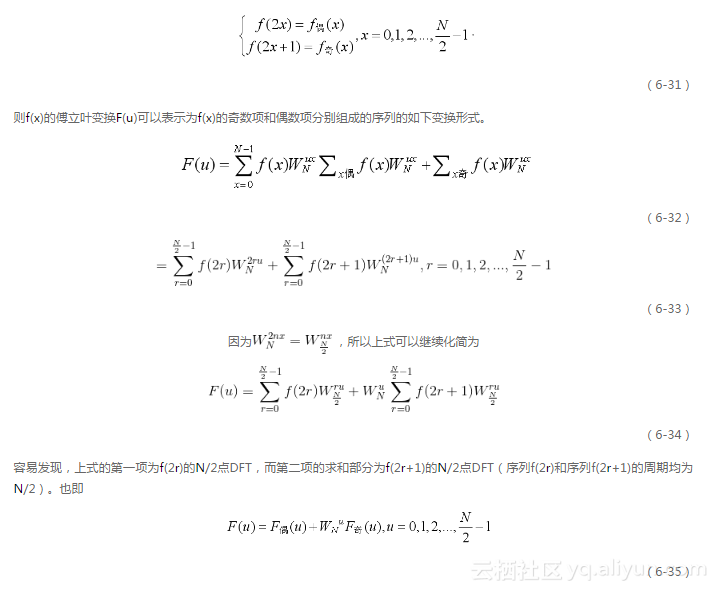这里，我们用F 偶(u) 和F 奇(u) 分别表示f(2r)和f(2r+1)的N/2点DFT。
而且，根据DFT序列的周期性特点，还可得到如下式子成立。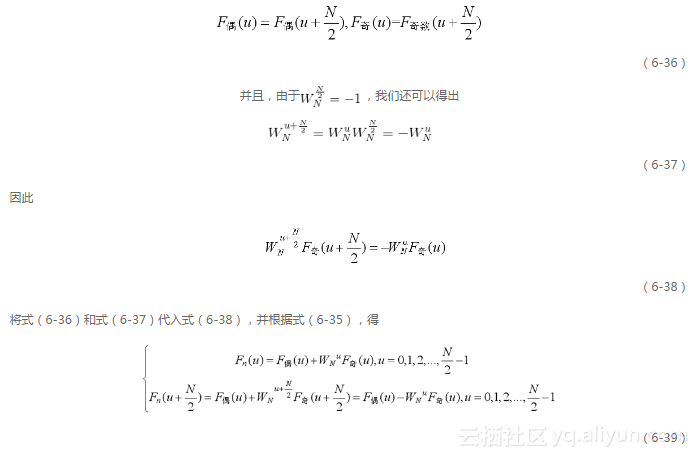这是一个递推公式，它就是FFT蝶形运算的理论依据。该公式表明，一个偶数长度序列的傅立叶变换可以通过它的奇数项和偶数项的傅立叶变换得到，从而可以将输入序列分成两部分分别计算，并按公式相加/相减。而在这个运算过程中，实际上只需要计算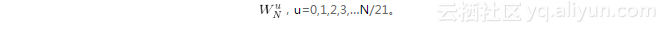因此，一个8点按时间抽取的FFT算法的第一步骤如图6.7所示。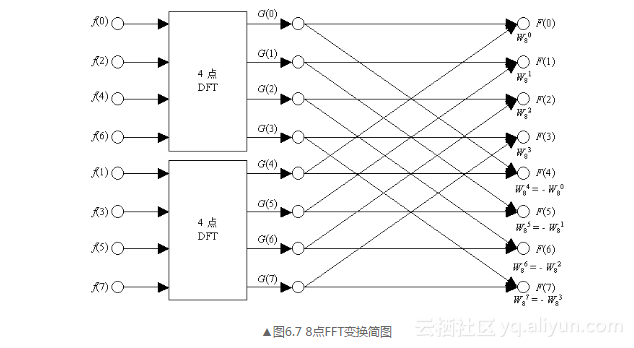图6.7所示是根据式（6-39）绘制的，这一算法也可以用图6.8抽象地表示出来。
由于我们讨论的是基-2的FFT算法，N/2 一般应是偶数，因此得到的序列还可以继续分解，分解过程可以一直持续到每个序列只需要2点的DFT。这样只需要如下的运算即可计算这一DFT值，这一运算是FFT的基本运算，称为蝶形运算。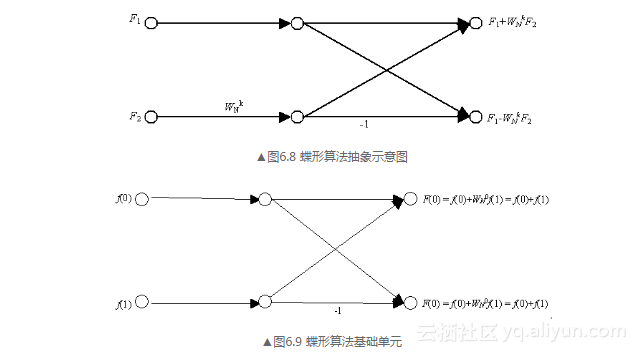这一基础单元是对初始输入序列进行傅立叶变换操作的第一步，即2点时的FFT。把这个基本的DFT运算和上面的抽象化蝶形运算比较，可以发现它们的基本结构是完全一致的。在蝶形算法中，我们可以只计算一次 W_N^k  F 2，而后分别与F 1相加和相减，从而每一次蝶形算法只需1次复数乘法和2次复数加法（从复杂度分析的角度，相减当然也可看作是一次加法）。并且，注意到 W_N^K =1，因此可以进一步简化计算。尤其第一级蝶形运算更是可以完全简化为单纯的复数加减法。
一个8点FFT的完整计算过程如图6.10所示，请思考这个过程与DFT过程的区别，以及这个过程所需的算法复杂度和存储空间问题。稍后我们将讨论这个问题。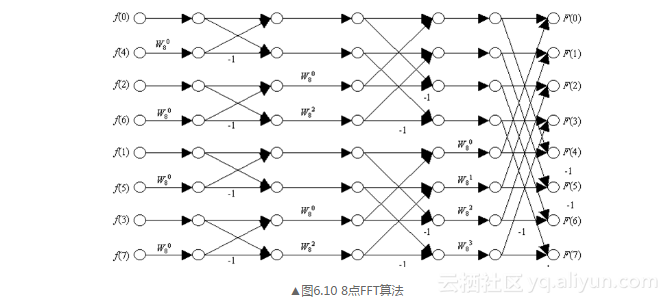用基-2的时间抽取FFT算法比直接计算DFT的效率高得多。在计算长度为N=2L序列的FFT时，在不对复数乘法进行额外优化的情况下，所需运算量分析如下。
对于每一个蝶形运算，我们需要进行1次复数乘法和2次复数加法。而FFT运算的每一级都含有N/2 = 2L-1个蝶形运算单元。因此，完成L级FFT运算共需要的复数乘法次数M cm和复数加法M ca数目分别为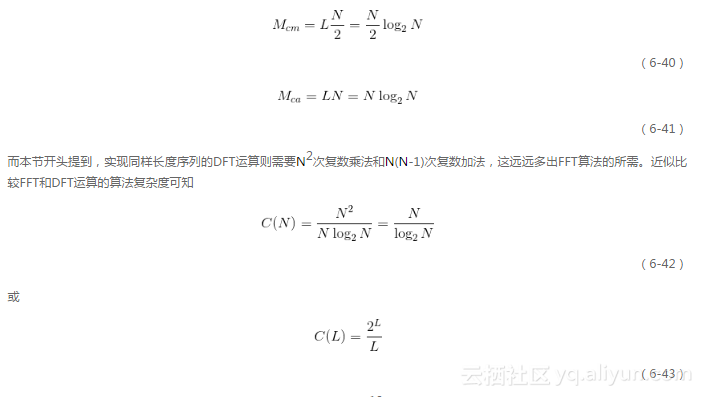因此，在N或L取值增大时，FFT运算的优势更加明显。例如，当N=210时，C(N)=102.4，即FFT算法的速度是DFT的102.4倍。
此外，从占用的存储空间看，按时间抽取的FFT算法也远比DFT算法节约。一对复数进行完蝶形运算后，就没有必要再次保留输入的复数对。因此，输出对可以和输入对放在相同的存储单元中。所以，只需要和输入序列大小相等的存储单元即可。也就是一种“原位运算”。
但是，经过观察上面的8点FFT运算全过程，我们发现，如果要使用这种“原位”运算，输入序列就必须按照倒序存储。由于f(x)是逐次抽取的，所以必须对原输入码列倒转位序，得到的次序相当于是原序列编号的二进制码位倒置。也即将原序列编号按照二进制表示，并且将二进制的所有位次序颠倒，就得到了在实际的输入序列中应该使用的排序位置。下面同样以8点FFT为例说明码位倒置的方法，如表6.1所示。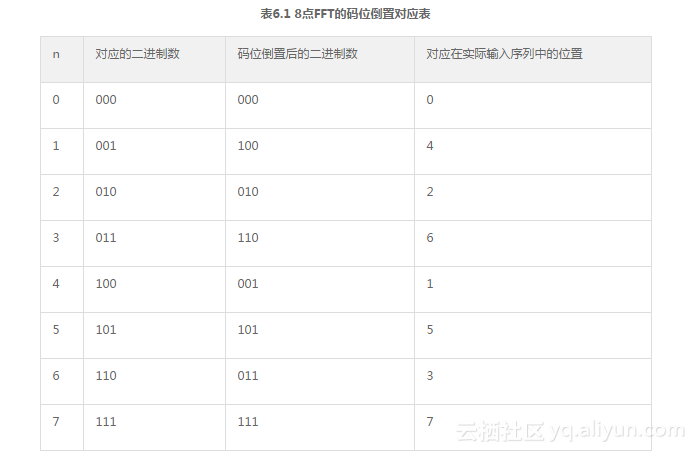按照表6.1中的顺序排列输入数据，就可以方便地进行原位运算，以节约内存空间。
6.3.4 离散反傅立叶变换的快速算法
离散反傅立叶变换的形式与离散傅立叶变换很相似,首先比较它们的公式形式。
离散反傅立叶变换IDFT的公式为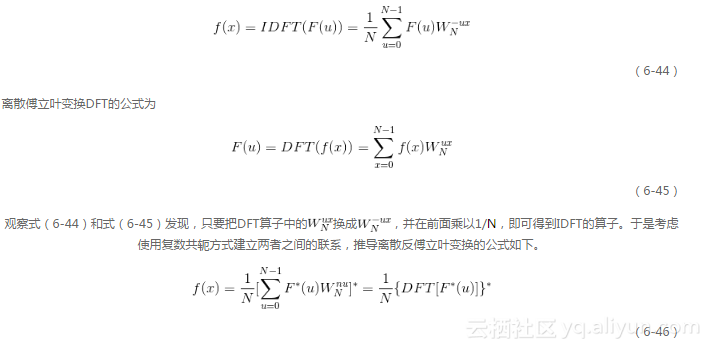因此，我们只需先将F(u)取共轭，就可以直接使用FFT算法计算IFFT了。
6.3.5 N维快速傅立叶变换N维快速傅立叶变换用于对高维信号矩阵执行傅立叶频谱分析操作。其中二维的快速傅立叶变换常常用于数字图像处理。
N维快速傅立叶变换是由一维FFT组合而成的，其运算实质就是在给定二维或多维数组的每个维度上依次执行一维FFT，并且使用“原位运算”的方法。在开始之前，算法将输入直接复制到输出上，所以之后在每个维度上执行FFT的原位操作都不会改变原本的输入数组，同时也使这个算法输出的数组和输入的数组拥有同样的大小和维度。也就是说，如果对一幅灰度图像执行二维快速傅立叶变换操作，得到的结果也将是一个二维数组。
6.3.6 Matlab实现
Matlab中提供了fft2和ifft2函数分别计算二维傅立叶变换和反变换，它们都经过了优化，运算速度非常快；另一个与傅立叶变换密切相关的函数是fftshift，常需要利用它来将傅立叶频谱图中的零频点移动到频谱图的中心位置。
下面分别介绍这3个函数。
1．fft2函数该函数用于执行二维快速傅立叶操作，因此可以直接用于数字图像处理。调用语法为
Y = fft2(X)
Y = fft2(X,m,n)

参数说明
X为输入图像。m和n分别用于将X的第一和第二维规整到指定的长度。当m和n均为2的整数次幂时，算法的执行速度要比m和n均为素数时更快。返回值
Y是计算得到的傅立叶频谱，是一个复数矩阵。提示
计算abs(Y )可得到幅度谱，计算angle(Y )可得到相位谱。2．fftshift函数在fft2函数输出的频谱分析数据中，是按照原始计算所得的顺序来排列频谱的，而没有以零频为中心来排列，因此造成了零频在输频谱矩阵的角上，显示幅度谱图像时表现为4个亮度较高的角（零频处的幅值较高），如图6.11!（a）所示。image
fftshift函数利用了频谱的周期性特点，将输出图像的一半平移到另一端，从而使零频被移动到图像的中间。其调用语法为
Y = fftshift(X)
Y = fftshift(X,dim)

参数说明
X为要平移的频谱。dim指出了在多维数组的哪个维度上执行平移操作。返回值
Y是经过平移的频谱。利用fftshift函数对图6.11（a）中的图像平移后的效果如图6.11（b）所示。
下面给出对于二维图像矩阵，fftshift函数的平移过程，如图6.12所示。
可见，输出矩阵被分为了4个部分，其中1、3两部分对换，2、4两部分对换。这样，原来在角上的零频率点（原点）位置就移动到了图像的中央位置。而dim参数则可以指定在多维数组的哪个维度上执行对换操作。例如，对于矩阵而言，dim取1和2的情形分别如图6.13所示。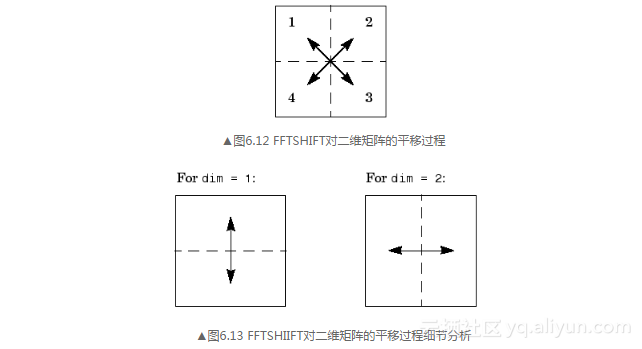3．ifft2函数该函数用于对图像（矩阵）执行逆傅立叶变换。输出矩阵的大小与输入矩阵相同。调用形式为
Y = ifft2(X)
Y = ifft2(X,m,n)

参数说明
X为要计算反变换的频谱。m、n的意义与fft2中相同。返回值
Y是反变换后得到的原始图像。注意
在执行IFFT2函数之前，如果曾经使用FFTSHIFT函数对频域图像进行过原点平移，则还需要使用IFFTSHIFT将原点平移回原位置。[例6.1] 幅度谱的意义
下面的程序展示了如何利用fft2进行二维快速傅立叶变换。为了更好地显示频谱图像，需要利用3.3节中学习过的对数变换来增强频谱。
I1 = imread('cell.tif');    %读入原图像
fcoef = fft2(double(I1));   %做fft变换
spectrum = fftshift(fcoef);  %将零点移到中心
temp =log(1+abs(spectrum));  %对幅值做对数变换以压缩动态范围

subplot(1,2,1);
imshow(temp,[]);
title('FFT');
subplot(1,2,2);
imshow(I1);
title('Source')

fcoef = fft2(double(I2));   %做fft变换
spectrum = fftshift(fcoef);  %将零点移到中心
temp =log(1+abs(spectrum));  %对幅值做对数变换以压缩动态范围

figure;
subplot(1,2,1);
imshow(temp,[]);
title('FFT');
subplot(1,2,2);
imshow(I2);
title('Source')

上述程序的运行结果如下。
由图6.14所示可以看出，图6.14（b）中的cell.tif图像较为平滑，而在其傅立叶频谱中，低频部分对应的幅值较大；而对图6.14（d）中细节复杂的的图像circuit.tif，灰度的变化趋势更加剧烈，相应的频谱中高频分量较强。
事实上，由于图6.14（b）图中基本只存在水平和垂直的线条，导致了在输出的频谱中亮线集中存在于水平和垂直方向（并且经过原点）。具体地说，原图像中的水平边缘对应于频谱中的竖直亮线，而竖直边缘则对应着频谱中的水平响应。我们不妨这样理解，水平方向的边缘可以看作在竖直方向上的灰度值的矩形脉冲，而这样的矩形脉冲可以分解为无数个竖直方向正弦平面波的叠加，从而对应频域图像中的垂直亮线；而对于竖直方向的边缘，情况是类似的。
通过例6.1可以发现一些频谱与其空间域图像之间的联系。实际上，低频（频谱图像中靠近中心的区域）对应着图像的慢变化分量；高频（频谱图像中远离中心的区域）对应着一幅图像中较快变化的灰度级，常常对应着图像细节，如物体的边缘和噪声等。就拿图6.14（c）的电路图像来说，电路板的灰度较为一致的背景区域就对应着频谱的低频部分，而横竖电路线条的灰度变换则是相对高频的成份，且灰度变换越剧烈，就对应着越高的频域分量。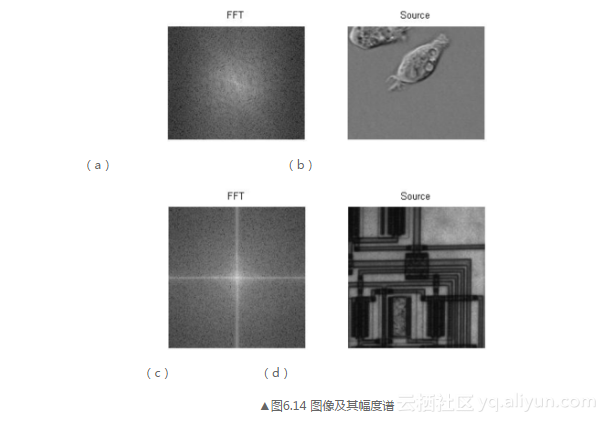我们在6.2.3小节曾给出了幅度谱和相位谱的定义，并对其作用进行了简单的介绍。为了进一步加深读者对幅度谱和相位谱的认识，这里给出一个关于它们的有趣的例子。
[例6.2]美女与猫——交换两幅图像的相位谱
图6.15（a）、（b）中分别是一张美女的照片和一张猫的照片，这里我们准备交换这两幅图像的相位谱，即用美女的幅度谱加上猫的相位谱，而用猫的幅度谱加上美女的相位谱，然后根据式（6-18），通过幅度谱和相位谱来还原傅立叶变换F(u,v)，再经傅立叶反变换得到交叉相位谱之后的图像。根据6.2.2中关于幅度谱和相位谱各自作用的讨论，您能想到这样做将会产生怎样的结果吗?
% ex6_2.m

% 读取图片

% 求傅立叶变换
Af = fft2(double(A));
Bf = fft2(double(B));

% 分别求幅度谱和相位谱
AfA = abs(Af);
AfB = angle(Af);

BfA = abs(Bf);
BfB = angle(Bf);

% 交换相位谱并重建复数矩阵
AfR = AfA .* cos(BfB) + AfA .* sin(BfB) .* i;
BfR = BfA .* cos(AfB) + BfA .* sin(AfB) .* i;

% 傅立叶反变换
AR = abs(ifft2(AfR));
BR = abs(ifft2(BfR));

% 显示图像
subplot(2,2,1);
imshow(A);
title('美女原图像');

subplot(2,2,2);
imshow(B);
title('猫的原图像');

subplot(2,2,3);
imshow(AR, []);
title('美女的幅度谱和猫的相位谱组合');

subplot(2,2,4);
imshow(BR, []);
title('猫的幅度谱和美女的相位谱组合');

程序运行结果如图6.15所示。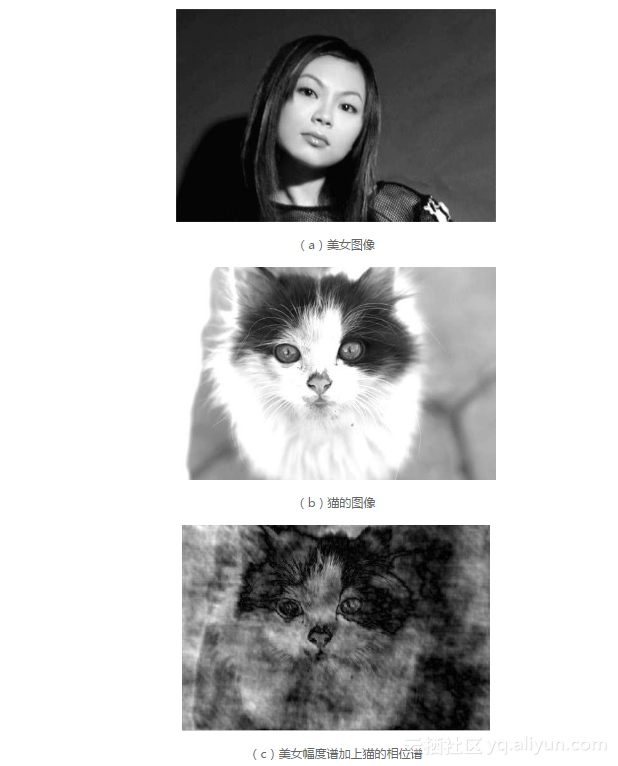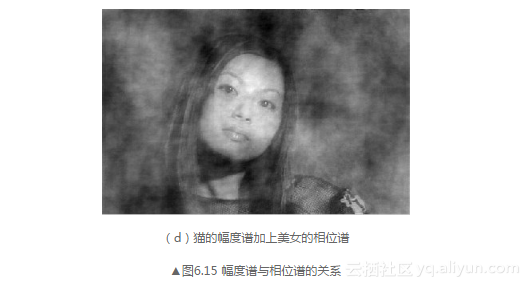通过这个示例，我们可以发现，交换相位谱之后，反变换之后得到的图像内容与其相位谱对应的图像一致，这就印证了我们之前关于相位谱决定图像结构的论断。而图像中整体灰度分布的特性，如明暗、灰度变化趋势等则在比较大的程度上取决于对应的幅度谱，因为幅度谱反映了图像整体上各个方向的频率分量的相对强度。


展开全文• 显示索引图像和灰度图像&gt;&gt; [X,map]=imread('trees.tif');&gt;&gt; gmap=rgb2gray(map);&gt;&gt; figure,imshow(X,map);&gt;&gt; figure,imshow(X,gmap);利用膨胀函数平移图像...matlab函数 数字图像处理函数
• 平滑滤波——matlab图像处理 平滑滤波的目的是消除或尽量减少噪声，改善图像的质量。假设加性噪声是随机独立分布，这样利用图像像素领域的平均或加权平均即可有效地抑制噪声干扰。从信号分析的观点来看，图像平滑...平滑滤波 Matlab
• MATLAB中，基本数据结构为数列，大部分...这样就把图像变成了数列了，MATLAB就可以使用数学函数对图像进行处理了。 1.MATLAB图像表达方式 在MATLAB中，图像可以以两种方式表达，分别为像素索引和空间位置。 （1）
• MATLAB–数字图像处理–图像的分类 图像的分类 图像的属性是多角度的，图像的分类也是多维的。 根据数字图像在计算机中表示方法的不同，分为二值图像、灰度图像、RGB图像和索引图像。 二值图像 二值图像(Binary ...matlab
• 使用matlab 读取Lena.bmp图像，并将其显示为： 1、原始图像 2、左右翻转的图像 3、上下翻转的图像 4、放大一倍的图像 5、显示其直方图 6、图像向右下方平移半个图像长宽的距离 没有以分号结尾的话就会显示...
• 基于matlab图像处理，概述如何应用该软件实现对车辆的监测和识别matlab 图像处理
• 表1 图像显示 函数名 功能说明 函数名 功能说明 colorbar 颜色条显示 montage 按矩形剪辑方式显示多帧图像 getimage 从坐标...
• 本节书摘来自异步社区出版社《MATLAB图像处理超级学习手册》一书中的第1章，第1.1节，作者：MATLAB技术联盟 , 张岩 更多章节内容可以访问云栖社区“异步社区”公众号查看。 1.1 MATLAB简介 MATLAB图像处理超级学习...
• 经常做做图像处理的东西，时间长了，有些函数就忘了，看到网上有人总结，收藏了。 1. 图像和图像数据  缺省情况下，MATLAB将图像中的数据存储为双精度类型(double)，64位浮点数，所需存储量很大；MATLAB还...matlab 图像处理
• Matlab计算机视觉/图像处理工具箱推荐 2014年4月9日机器学习MATLAB, 计算机视觉luffylee 计算机视觉/图像处理研究中经常要用到Matlab，虽然其自带了图像处理和计算机视觉的许多功能，但是术业有专攻，在进行...
• MATLAB图像处理常用函数 作者：陈若愚 日期：2020年2月28日 QQ：2389388826 一、基本操作： 1. 显示索引图像和灰度图像 [X,map]=imread('trees.tif'); gmap=rgb2gray(map); figure,imshow(X,map); figure,imshow(X...matlab 图像处理
• 图像处理工具箱引用图像处理工具箱1. 图像和图像数据  缺省情况下，MATLAB将图像中的数据存储为双精度类型(double)，64位浮点数，所需存储量很大；MATLAB还支持另一种类型无符号整型(uint8)，即图像矩阵中每个数据...matlab 图像处理 工具 filter
• 维纳滤波复原 x = deconvwnr(I,PSF,SNR); PSF为点扩展函数，SNR为信噪比。 fspecial:创造一个2维滤波器，LEN为运动长度（模糊程度），THETA为运动角度 imfilter：对多维图像进行N-D滤波 ...matlab
...